# Solving radical equations

##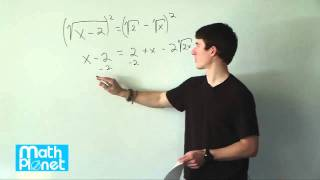By MathPlanetVideos

Solve the following radical expression x−2−−−−√=2–√−x−−√# Adding and Subtracting Radicals

##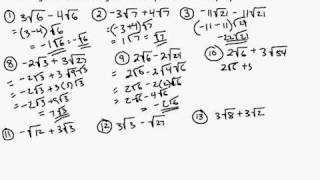By MrLovellFord

This algebra video offers more information on adding and subtracting radicals, and includes a sample walkthrough.# Simplifying Radicals With Variables, Exponents, Fractions, Cube Roots - Algebra

##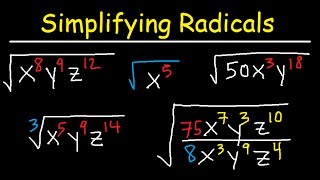By The Organic Chemistry Tutor

This algebra 1 & 2 video tutorial shows you how to simplify radicals with variables, fractions, and exponents that contains both square roots, cube roots, and variables such as x, y, and z. This video contains plenty of examples and practice problems.Here is a list of topics: 1. Simplifying Radical Expressions With Variables & Exponents 2. Simplifying Radicals With Fractions & Variables 3. Radicals With Square Roots & Cube Roots 4. Radical Expressions with X, Y, and Z 5. Absolute Value - Simplifying radical expressions# Unit-fraction exponents and radicals

##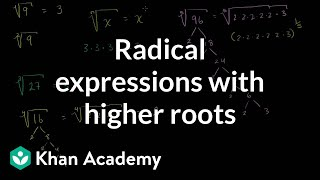By Khan Academy

Sal explains the relationship between radical notation and unit-fraction exponents, including a discussion of what the principal square root is. Then he shows how to simplify the 6th root of (64x^8) using the properties of exponents.# Radical equivalent to rational exponents | Algebra I | Khan Academy

##By Khan Academy

Radical Equivalent to Rational Exponents# Ex: Evaluate an Expression with Rational Exponents Using Radicals

##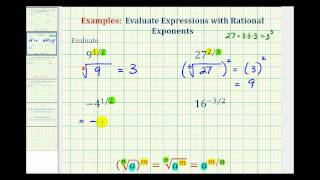By Mathispower4u

This video provides examples of how to evaluate an expressions with rational exponents by writing the expression in radical form and then simplifying.# Simplifying radicals | Exponents, radicals, and scientific notation | Pre-Algebra | Khan Academy

##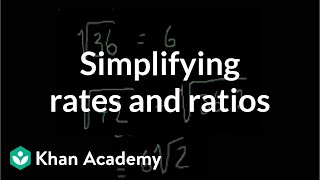By Khan Academy

This video lecture series on Pre-algebra from Khan Academy includes Order of Operations, Adding and Subtracting Negative Numbers, Multiplying and Dividing Negative Numbers, Adding and Subtracting Fractions, Multiplying and Dividing Fractions, Exponents, Exponent Rules, Simplifying Radicals, Introduction to Logarithms, Unit Conversion, Speed translation.# Free Radical Reactions

##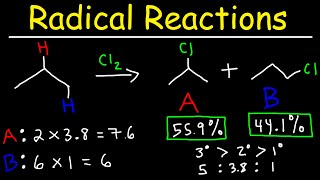By The Organic Chemistry Tutor

This video provides a basic introduction into free radical reactions. It explains the reactivit-selectivity principle between Chlorine and Bromine and it explains how to calculate the relative percent yields of each monochlorinated product. It discusses heterolytic and homolytic cleavage as it relates to the formation of radicals as well as how to identify initiation, propagation and termination steps in a radical reaction.# Simplify numerical radical expressions with multiple terms

##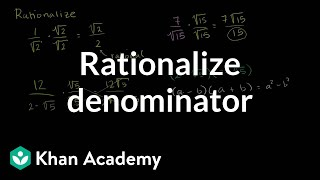By Khan Academy

Sal shows how to manipulate expressions like 1/2 and 12/(2-5) so there's no radical in the denominator. This is called rationalizing the denominator.45# Radical equivalent to rational exponents 2 | Algebra I | Khan Academy

##By Khan Academy

Khan Academy presents Radical Equivalent to Rational Exponents 2, an educational video resource on math.# Add, subtract, multiply, and divide numerical radical terms

##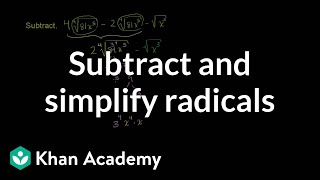By Khan Academy

Sal shows how to group like terms to subtract and simplify radical terms, by simplifying the expression 4ï¿½ï¿½ï¿½ï¿½ï¿½ï¿½ï¿½ï¿½ï¿½ï¿½ï¿½ï¿½ï¿½ï¿½ï¿½ï¿½ï¿½ï¿½(81x^5)-2ï¿½ï¿½ï¿½ï¿½ï¿½ï¿½ï¿½ï¿½ï¿½ï¿½ï¿½ï¿½ï¿½ï¿½ï¿½ï¿½ï¿½ï¿½(81x^5)-ï¿½ï¿½ï¿½ï¿½ï¿½ï¿½ï¿½ï¿½ï¿½ï¿½ï¿½ï¿½ï¿½ï¿½ï¿½ï¿½ï¿½ï¿½(x^3).# Radical equations

##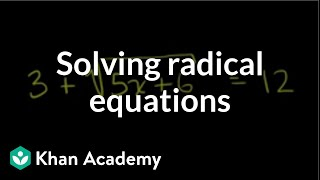By Khan Academy

Sal solves the equationï¿½ï¿½ï¿½ï¿½ï¿½ï¿½ï¿½ï¿½ï¿½ï¿½ï¿½ï¿½3+ï¿½ï¿½ï¿½ï¿½ï¿½ï¿½ï¿½ï¿½ï¿½ï¿½ï¿½ï¿½ï¿½ï¿½ï¿½ï¿½ï¿½ï¿½(5x+6)=12.# Radical equations

##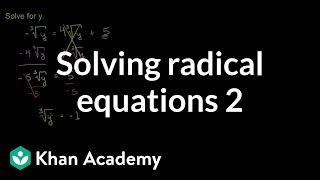By Khan Academy

Sal solves the equationï¿½ï¿½ï¿½ï¿½ï¿½ï¿½ï¿½ï¿½ï¿½ï¿½ï¿½ï¿½-ï¿½ï¿½ï¿½ï¿½ï¿½ï¿½ï¿½ï¿½ï¿½ï¿½ï¿½ï¿½ï¿½ï¿½ï¿½ï¿½ï¿½ï¿½ï¿½ï¿½ï¿½y=4ï¿½ï¿½ï¿½ï¿½ï¿½ï¿½ï¿½ï¿½ï¿½ï¿½ï¿½ï¿½ï¿½ï¿½ï¿½ï¿½ï¿½ï¿½ï¿½ï¿½ï¿½y+5.# Radical equations

##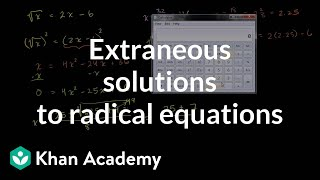By Khan Academy

Sal explains what square-root equations are, and shows an example of solving such an equation and checking for extraneous solutions.# Radical equations

##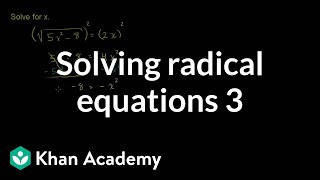By Khan Academy

Sal solves the equationï¿½ï¿½ï¿½ï¿½ï¿½ï¿½ï¿½ï¿½ï¿½ï¿½ï¿½ï¿½ï¿½ï¿½ï¿½ï¿½ï¿½ï¿½ï¿½ï¿½ï¿½ï¿½ï¿½ï¿½ï¿½ï¿½ï¿½ï¿½ï¿½ï¿½(5x^2-8)=2x.# Solving radical equations 1 | Exponent expressions and equations | Algebra I | Khan Academy

##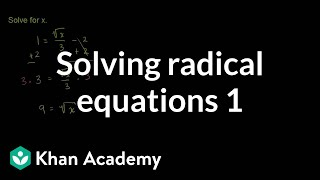By Khan Academy

This Khan Academy video offers more information on solving radical equations and contains a few sample problems with a step-by-step walkthrough on solving these equations.# Add, subtract, multiply, and divide numerical radical terms

##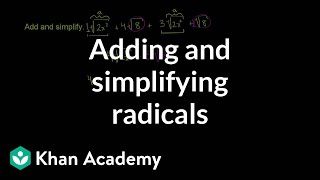By Khan Academy

Sal shows how to group like terms to add and simplify radical terms, by simplifying the expression ï¿½ï¿½ï¿½ï¿½ï¿½ï¿½ï¿½ï¿½ï¿½ï¿½ï¿½ï¿½ï¿½ï¿½ï¿½ï¿½ï¿½ï¿½(2x^2)+4ï¿½ï¿½ï¿½ï¿½ï¿½ï¿½ï¿½ï¿½ï¿½ï¿½ï¿½ï¿½ï¿½ï¿½ï¿½ï¿½ï¿½ï¿½8+3ï¿½ï¿½ï¿½ï¿½ï¿½ï¿½ï¿½ï¿½ï¿½ï¿½ï¿½ï¿½ï¿½ï¿½ï¿½ï¿½ï¿½ï¿½(2x^2)+ï¿½ï¿½ï¿½ï¿½ï¿½ï¿½ï¿½ï¿½ï¿½ï¿½ï¿½ï¿½ï¿½ï¿½ï¿½ï¿½ï¿½ï¿½8.# Unit-fraction exponents and radicals

##By Khan Academy

Sal explains what it means to take a number by a power which is a unit fraction, like 1/2, 1/3, etc.# Unit-fraction exponents and radicals

##By Khan Academy

Sal explains the relationship between radical notation and unit-fraction exponents, including a discussion of what the principal square root is. Then he shows how to simplify the 6th root of (64x^8) using the properties of exponents.# Single-step simplification of radical expressions

##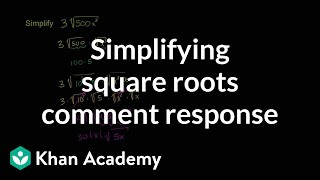By Khan Academy

Sal shows how to simplify 3ï¿½ï¿½ï¿½ï¿½ï¿½ï¿½ï¿½ï¿½ï¿½ï¿½ï¿½ï¿½ï¿½ï¿½ï¿½ï¿½ï¿½ï¿½(500x^3).